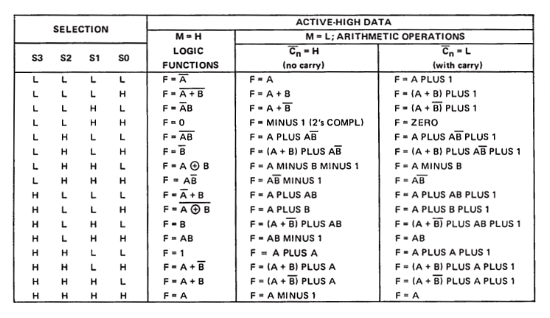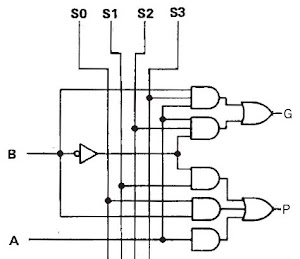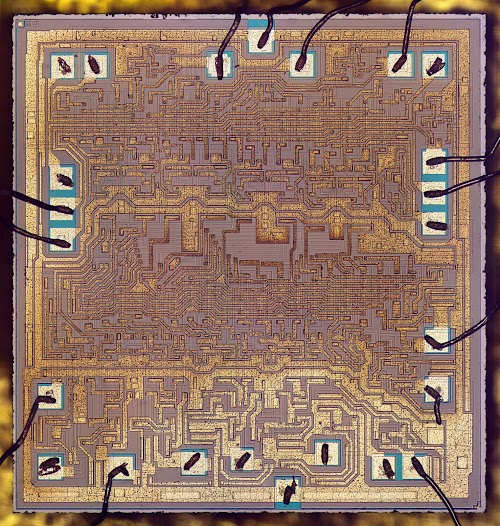### Inside the vintage 74181 ALU chip: how it works and why it's so strange

The 74181 ALU (arithmetic/logic unit) chip powered many of the minicomputers of the 1970s: it provided fast 4-bit arithmetic and logic functions, and could be combined to handle larger words, making it a key part of many CPUs. But if you look at the chip more closely, there are a few mysteries. It implements addition, subtraction, and the Boolean functions you'd expect, but why does it provide several bizarre functions such as "A plus (A and not B)"? And if you look at the circuit diagram (below), why does it look like a random pile of gates rather than being built from standard full adder circuits. In this article, I explain that the 74181's set of functions isn't arbitrary but has a logical explanation. And I show how the 74181 implements carry lookahead for high speed, resulting in its complex gate structure.Schematic of the 74LS181 ALU chip, from the datasheet. The internal structure of the chip is surprisingly complex and difficult to understand at first.

The 74181 chip is important because of its key role in minicomputer history. Before the microprocessor era, minicomputers built their processors from boards of individual chips. A key part of the processor was the arithmetic/logic unit (ALU), which performed arithmetic operations (addition, subtraction) and logical operations (AND, OR, XOR). Early minicomputers built ALUs out of a large number of simple gates. But in March 1970, Texas Instruments introduced the 74181 Arithmetic / Logic Unit (ALU) chip, which put a full 4-bit ALU on one fast TTL chip. This chip provided 32 arithmetic and logic functions, as well as carry lookahead for high performance. Using the 74181 chip simplified the design of a minicomputer processor and made it more compact, so it was used in many minicomputers. Computers using the 74181 ranged from the popular PDP-11 and Xerox Alto minicomputers to the powerful VAX-11/780 "superminicomputer". The 74181 is still used today in retro hacker projects.1

The 74181 implements a 4-bit ALU providing 16 logic functions and 16 arithmetic functions, as the datasheet (below) shows. As well as the expected addition, subtraction, and Boolean operations, there are some bizarre functions such as "(A + B) PLUS AB".The datasheet for the 74181 ALU chip shows a strange variety of operations.

So how is the 74181 implemented and why does it include such strange operations? Is there any reason behind the 74181's operations, or did they just randomly throw things in? And why are the logic functions and arithmetic functions in any particular row apparently unrelated? I investigated the chip to find out.

## The 16 Boolean logic functions

There's actually a system behind the 74181's set of functions: the logic functions are the 16 possible Boolean functions f(A,B). Why are there 16 possible functions? If you have a Boolean function f(A,B) on one-bit inputs, there are 4 rows in the truth table. Each row can output 0 or 1. So there are 2^4 = 16 possible functions. Extend these to 4 bits, and these are exactly the 16 logic functions of the 74181, from trivial 0 and 1 to expected logic like A AND B to contrived operations like NOT A AND B. These 16 functions are selected by the S0-S3 select inputs.

## Arithmetic functions

The 74181's arithmetic operations are a combination of addition, subtraction, logic operations, and strange combinations such as "A PLUS AB PLUS 1". It turns out that there is a rational system behind the operation set: they are simply the 16 logic functions added to A along with the carry-in.2 That is, the arithmetic functions are: A PLUS f(A,B) PLUS carry-in. For example, If f(A,B)=B, you get simple addition: A PLUS B PLUS carry-in. If f(A,B) = NOT B, you get A PLUS NOT B PLUS carry-in, which in two's-complement logic turns into subtraction: A MINUS B MINUS 1 PLUS carry-in.

Other arithmetic functions take a bit more analysis. Suppose f(A,B) = NOT (A OR B). Then each bit of A PLUS f(A,B) will always be 1 except in the case where A is 0 and B is 1, so the result of the sum is A OR NOT B. Even though you're doing addition, the result is a logical function since no carry can be generated. The other strange arithmetic functions can be understood similarly.3

Thus, the 16 arithmetic functions of the 74181 are a consequence of combining addition with one of the 16 Boolean functions. Even though many of the functions are strange and probably useless, there's a reason for them. (The Boolean logic functions for arithmetic are in a different order than for logical operations, explaining why there's no obvious connection between the arithmetic and logical functions.)

Carry lookahead uses "Generate" and "Propagate" signals to determine if each bit position will always generate a carry or can potentially generate a carry. For instance, if you're adding 0+0+C (where C is the carry-in), there's no way to get a carry out from that addition, regardless of what C is. On the other hand, if you're adding 1+1+C, there will always be a carry out generated, regardless of C. This is called the Generate case. Finally, for 0+1+C (or 1+0+C), there will be a carry out if there is a carry in. This is called the Propagate case since if there is a carry-in, it is propagated to the carry out.4 Putting this all together, for each bit position you create a G (generate) signal if both bits are 1, and a P (propagate) signal unless both bits are 0.

The carry from each bit position can be computed from the P and G signals by determining which combinations can produce a carry. For instance, there will be a carry from bit 0 to bit 1 if P0 is set (i.e. a carry is generated or propagated) and there is either a carry-in or a generated carry. So C1 = P0 AND (Cin OR G0).

Higher-order carries have more cases and are progressively more complicated. For example, consider the carry in to bit 2. First, P1 must be set for a carry out from bit 1. In addition, a carry either was generated by bit 1 or propagated from bit 0. Finally, the first carry must have come from somewhere: either carry-in, generated from bit 0 or generated from bit 1. Putting this all together produces the function used by the 74181: C2 = P1 AND (G1 OR P0) AND (C0 OR G0 OR G1).

As you can see, the carry logic gets more complicated for higher-order bits, but the point is that each carry can be computed from G and P terms and the carry-in. Thus, the carries can be computed in parallel, before the addition takes place.5

## Creating P and G with an arbitrary Boolean function

The previous section showed how the P (propagate) and G (generate) signals can be used when adding two values. The next step is to examine how P and G are created when adding an arbitrary Boolean function f(A, B), as in the 74181. The table below shows P and G when computing "A PLUS f(A,B)". For instance, when A=0 there can't be a Generate, and Propagate depends on the value of f. And when A=1, there must be a Propagate, while Generate depends on the value of f.

 A B A PLUS f(a,b) P G 0 0 0+f(0,0) f(0,0) 0 0 1 0+f(0,1) f(0,1) 0 1 0 1+f(1,0) 1 f(1,0) 1 1 1+f(1,1) 1 f(1,1)

In the 74181, the four f values are supplied directly by the four Select (S pin) values, resulting in the following table:6

 A B A PLUS f P G 0 0 0 S1 0 0 1 1 S0 0 1 0 1 1 S2 1 1 10 1 S3

The chip uses the logic block below (repeated four times) to compute P and G for each bit. It is straightforward to verify that it implements the table above. For instance, G will be set if A is 1, B is 1 and S3 is 1, or if A is 1, B is 0 and S2 is set.This circuit computes the G (generate) and P (propagate) signals for each bit of the 74181 ALU chip's sum. The S0-S3 selection lines select which function is added to A.

## Creating the arithmetic outputs

The addition outputs are generated from the internal carries (C0 through C3), combined with the P and G signals. For each bit, A PLUS f is the same as P ⊕ G, so adding in the carry gives us the full 4-bit sum. Thus, F0 = C0 ⊕ P0 ⊕ G0, and similarly for the other F outputs.7 On the schematic, each output bit has two XOR gates for this computation.

## Creating the logic outputs

For the logic operations, the carries are disabled by forcing them all to 1. To select a logic operation, the M input is set to 1. M is fed into all the carry computation's AND-NOR gates, forcing the carries to 1. The output bit sum as as above, producing A ⊕ f ⊕ 1 = A ⊕ f. This expression yields all 16 Boolean functions, but in a scrambled order relative to the arithmetic functions.8

## Interactive 74181 viewer

To see how the circuits of the 74181 work together, try the interactive schematic below.9 The chip's inputs are along the top and right; click on any of them to change the value. The A and B signals are the two 4-bit arguments. The S bits on the right select the operation. C is the carry-in (which is inverted). M is the mode, 1 for logic operations and 0 for arithmetic operations. The dynamic chart under the schematic describes what operation is being performed.

The P and G signals are generated by the top part of the circuitry, as described above. Below this, the carry lookahead logic creates the carry (C) signals by combining the P and G signals with the carry-in (Cn). Finally, the sum for each bit is generated (Σ) from the P and G signals7, then combined with each carry to generate the F outputs in parallel.10

Result and truth table for inputs entered above
Select :

 0000 A 0000 B 0001 F 1001
 Ai Bi Pi Gi Fi 0 0 X Y F 0 1 X Y F 1 0 X Y F 1 1 X Y F

## Die photo of the 74181 chip.

I opened up a 74181, took die photos, and reverse engineered its TTL circuitry. My earlier article discusses the circuitry in detail, but I'll include a die photo here since it's a pretty chip. (Click image for full size.) Around the edges you can see the thin bond wires that connect the pads on the die to the external pins. The shiny golden regions are the metal layer, providing the chip's internal wiring. Underneath the metal, the purplish silicon is doped to form the transistors and resistors of the TTL circuits. The die layout closely matches the simulator schematic above, with inputs at the top and outputs at the bottom.Die photo of the 74181 ALU chip. The metal layer of the die is visible; the silicon (forming transistors and resistors) is hidden behind it.

## Conclusion

While the 74181 appears at first to be a bunch of gates randomly thrown together to yield bizarre functions, studying it shows that there is a system to its function set: it provides all 16 Boolean logic functions, as well as addition to these functions. The circuitry is designed around carry lookahead, generating G and P signals, so the result can be produced in parallel without waiting for carry propagation. Modern processors continue to use carry lookahead, but in more complex forms optimized for long words and efficient chip layout.12

I announce my latest blog posts on Twitter, so follow me at kenshirriff.

## Notes and references

1. Retro projects using the 74181 include the APOLLO181 CPU, Fourbit CPU, 4 Bit TTL CPU, Magic-1 (using the 74F381), TREX, Mark 1 FORTH and Big Mess o' Wires

2. The carry-in input and the carry-out output let you chain together multiple 74181 chips to add longer words. The simple solution is to ripple the carry from one chip to the next, and many minicomputers used this approach. A faster technique is to use a chip, the 74182 look-ahead carry generator, that performs carry lookahead across multiple 74181 chips, allowing them to all work in parallel.

3. One thing to note is A PLUS A gives you left shift, but there's no way to do right shift on the 74181 without additional circuitry.

4. To simplify the logic, the 74181 considers 1+1+C both a Propagate case and a Generate case. (Some carry lookahead systems consider 1+1+C to be a Propgate case but not a Generate case.) For the 74181's outputs, Propagate must be set for Generate to be meaningful.

5. The carry-lookahead logic in the 74181 is almost identical to the earlier 74LS83 adder chip. The 74181's circuitry can be viewed as an extension of the 74LS83 to support 16 Boolean functions and to support logical functions by disabling the carry.

6. The way the S0 and S1 values appear in the truth table seems backwards to me, but that's how the chip works.

7. The bit sum Σ can be easily produced from the P and G signals. Some datasheets show each Σ signal generated as P XOR G, while other datasheets show Σ generated as NOT P AND G. Since the combination P=0, G=1 never arises, both generate the same results. I show XOR on the schematic as it is conceptually easier to understand, but examining the die shows the physical circuit uses the NOT/AND gates.

8. The logic functions are defined in terms of Select inputs as follows:

 A B F 0 0 S1 0 0 S0 0 0 S2 0 0 S3
Because the first two terms are inverted, the logic function for a particular select input doesn't match the arithmetic function.

9. The schematic is based on a diagram by Poil on WikiMedia, CC By-SA 3.0, with circuitry and labeling changes.

10. The 74181 chip has a few additional outputs. The A=B output is used with the subtraction operation to test the two inputs for equality. The Cn+4 output is the inverted carry out, supporting longer words. P and G are the carry propagate and generate outputs, used for carry lookahead with longer words.11

11. The P and G outputs in my schematic are reversed compared to the datasheet, for slightly complicated reasons. I'm describing the 74181 with active-high logic, where a high signal indicates 1, as you'd expect. However, the 74181 can also be used with active-low logic, where a low signal indicates a 1. The 74181 works fine with active-low logic except the meanings of some pins change, and the operations are shuffled around. The P and G labels on the datasheet are for active-low logic, so with active-high, they are reversed.Anonymous said...

I seem to remember some similar stuff in the high loop of the IFR service monitor, the 1200, 500 and 1500 had the same one I think. This was a unit that generated RF in coarse steps between 2 and 3 GHz, iirc, and was implemented as two boards in a module packed with ECL ICs. I'd never seen ECL before and if i have since don't remember it.

That would have been a box you would have loved, the IFR service monitor.Len said...

It looks like this ALU (or related) was used in at least one arcade machine in 1980 - as part of a 12-bit processor - by a company named Cinematronics:

Unknown said...

There is another explanation of the '181 here:
http://web.eecs.umich.edu/~jhayes/iscas.restore/74181.htmlAnonymous said...

Why do s0 and s1 seem backwards? They are in the standard order they should be, counting up in binary.

Daniel

Unknown said...

For your discussion of carry lookahead, I'm assuming C0 = Cin. Is that right?

Joe Zbiciak said...

A later TI part, the TMS320C80 DSP, implemented a 3-operand boolean unit with selectable carry chain. You could provide an arbitrary 3-operand truth table (8 bits), along with optionally selecting in the carry chain. This allowed for even more interesting hybrid operations such as (A + B) AND C.

Granted, the C80 was not a standalone ALU, and is quite a bit more obscure I imagine. But, it's the first thing I thought of when you started listing some of the curious functions the 74181 offers.

Unknown said...

Hey Ken!
Thanks for the great write-up! I've spent some time duplicating the block diagram with individual logic gates and have built up a couple of prototypes! let me know if you'd like for me to send you one!

thanks
Dave

Tony Cox said...

Comparing to the '181 datasheet (Nat Semi in my case) the logic diagram has the P and G outputs swapped compared to your lovely interactive graphic version. Which one is correct?

Paul said...

Hi Ken,

Great blog..brings me back quite a few years.

I can state with authority that the Prime (spelled Pr1me) computers that were TTL all used the 181 (what else would you do?)

That would be the P400, P750, P850 primarily.

The P9950 (which I worked on) was the first machine built from (10K) ECL and used the 10181. Fairly soon the LSI level bumped up and I never used them again.

-paul

Amaury said...

hi Ken,

this chip, with the 182, should be done in that google thing, probably in the fastest TTL-compatible process that can be manufactured (act ?)

https://fossi-foundation.org/2020/06/30/skywater-pdk